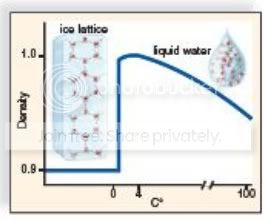# Biology Test 2 Part 2

11 Questions | Total Attempts: 268SettingsCreate your own QuizGoing over Biologys' 2nd test part 2

• 1.
In which of these are the electrons always shared unequally?
• A.

Double covalent bond

• B.

Polar covalent bond

• C.

Triple covalent bond

• D.

Ionic and covalent bonds

• E.

Hydrogen bond

• 2.
An example of a hydrogen bond would be the
• A.

Bond between a carbon atom and a hydrogen atom.

• B.

Bond between two carbon atoms.

• C.

Bond between sodium and chlorine.

• D.

Bond between two water molecules.

• 3.
Water flows freely, but does not separate into individual molecules because water is
• A.

Cohesive.

• B.

Hydrophobic.

• C.

Hydrophilic.

• D.

• 4.
Water can absorb a large amount of heat without much change in temperature, and therefore it has
• A.

A high heat capacity.

• B.

A high surface tension.

• C.

Ten times as many hydrogen ions.

• D.

Ten times as many hydroxide ions.

• 5.
Which of these properties of water cannot be attributed to hydrogen bonding between water molecules?
• A.

Water stabilizes temperature inside and outside the cell.

• B.

Water molecules are cohesive.

• C.

Water is a solvent for many molecules.

• D.

Ice floats on liquid water.

• E.

Water stablizes temperature inside and outside the cell. as well as Water is a solvent for many molecules

• 6.
This Graph illustrates
• A.

That ice is less dense than water.

• B.

That ice is more dense than water.

• C.

Why ice floats on water.

• D.

That ice is less dense than water.As well as Why Ice is more dense than water. are correct

• E.

Why water molecules form hydrogen bonds with other water molecules.

• F.

All of these are correct.

• 7.
Acids
• A.

Release hydrogen ions in solution.

• B.

Cause the pH of a solution to rise above 7.

• C.

Take up sodium ions and become neutral.

• D.

Increase the number of water molecules.

• E.

Release Hydrogen ions in solution and cause the Ph of a solution to rise above 7

• 8.
Which of these best describes the changes that occur when a solution goes from pH 5 to pH 8?
• A.

The hydrogen ion concentration decreases as the solution goes from acidic to basic

• B.

The hydrogen ion concentration increases as the solution goes from basic to acidic.

• C.

C. The hydrogen ion concentration decreases as the solution goes from basic to acidic.

• 9.
When water dissociates, it releases
• A.

Equal amounts of H+ and OH–.

• B.

b. more H+ than OH–.

• C.

C. more OH– than H+.

• D.

D. only H+.

• 10.
Rainwater has a pH of about 5.6; therefore, rainwater is
• A.

A neutral solution.

• B.

An acidic solution.

• C.

A basic solution.

• D.

It depends on whether the rainwater is buffered.

• 11.
If a chemical accepted H+ from the surrounding solution, the chemical could be
• A.

A base.

• B.

None of these are correct.

• C.

An acid.

• D.

A buffer.

• E.

A base and an acid

Related TopicsBack to top
×

Wait!
Here's an interesting quiz for you.